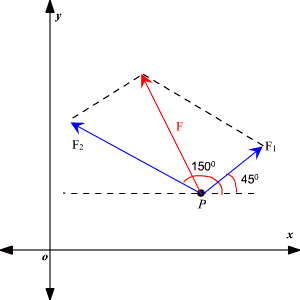# Solving Problems with Vectors

We can use vectors to solve many problems involving physical quantities such as velocity, speed, weight, work and so on.

### Velocity:

The velocity of moving object is modeled by a vector whose direction is the direction of motion and whose magnitude is the speed.

Example :

A ball is thrown with an initial velocity of $70$ feet per second., at an angle of $35°$ with the horizontal. Find the vertical and horizontal components of the velocity.

Let $\text{v}$ represent the velocity and use the given information to write $\text{v}$ in unit vector form:

$\text{v}\text{\hspace{0.17em}}=\text{70}\left(\mathrm{cos}\left(35°\right)\right)i+70\left(\mathrm{sin}\left(35°\right)\right)j$

Simplify the scalars, we get:

$\text{v}\text{\hspace{0.17em}}\approx 57.34i+40.15j$

Since the scalars are the horizontal and vertical components of $\text{v}$ ,

Therefore, the horizontal component is $57.34$ feet per second and the vertical component is $40.15$ feet per second.

### Force:

Force is also represented by vector. If several forces are acting on an object, the resultant force experienced by the object is the vector sum of these forces.

Example :

Two forces ${\text{F}}_{1}$ and ${\text{F}}_{2}$ with magnitudes $20$ and $30\text{\hspace{0.17em}}\text{lb}$ , respectively, act on an object at a point $\text{P}$ as shown. Find the resultant forces acting at $\text{P}$ .First we write ${\text{F}}_{1}$ and ${\text{F}}_{2}$ in component form:

$\text{v}\approx 57.34i+40.15j$

Simplify the scalars, we get:

$\begin{array}{l}\begin{array}{l}{\text{F}}_{1}=\left(20\mathrm{cos}\left(45°\right)\right)i+\left(20\mathrm{sin}\left(45°\right)\right)j\\ =20\left(\frac{\sqrt{2}}{2}\right)i+20\left(\frac{\sqrt{2}}{2}\right)j\end{array}\hfill \\ =10\sqrt{2}i+10\sqrt{2}j\hfill \\ \begin{array}{l}{\text{F}}_{2}=\left(30\mathrm{cos}\left(150°\right)\right)i+\left(30\mathrm{sin}\left(150°\right)\right)j\\ =30\left(-\frac{\sqrt{3}}{2}\right)i+30\left(\frac{1}{2}\right)j\end{array}\hfill \\ =-15\sqrt{3}i+15j\hfill \end{array}$

So, the resultant force $\text{F}$ is

$\begin{array}{l}\text{F}={\text{F}}_{1}+{\text{F}}_{2}\\ =\left(10\sqrt{2}\text{\hspace{0.17em}}i+10\sqrt{2}j\right)+\left(-15\sqrt{3}\text{\hspace{0.17em}}i+15j\right)\\ =\left(10\sqrt{2}-15\sqrt{3}\right)i+\left(10\sqrt{2}+15\right)j\\ \approx -12i+29j\end{array}$

### Work:

The work $\text{W}$ done by a force $\text{F}$ in moving along a vector $\text{D}$ is $\text{W}=\text{F}\cdot \text{D}$ .

Example :

A force is given by the vector $\text{F}=⟨2,3⟩$ and moves an object from the point $\left(1,3\right)$ to the point $\left(5,9\right)$ . Find the work done.

First we find the Displacement.

The displacement vector is

$\text{D}=⟨5-1,9-3⟩=⟨4,6⟩$ .

By using the formula, the work done is

$\text{W}=\text{F}\cdot \text{D}=⟨2,3⟩\cdot ⟨4,6⟩=26$

If the unit of force is pounds and the distance is measured in feet, then the work done is $26$ ft-lb.